• 差分进化算法（DE） MATLAB代码 差分进化算法（DE） MATLAB代码
• 差分进化算法Matlab代码，可运行，注释清楚 差分进化算法Matlab代码，可运行，注释清楚
• 利用差分演化算法求解函数的最小值，给出了十个实例函数。Matlab代码注释全
• 差分进化算法是模拟自然界生物种群以“优胜劣汰，适者生存”为原则的进化发展规律而形成的一种随机启发式搜索算法。其保留了基于种群的全局搜索策略，采用实数编码，基于差分的简单变异操作和一对一的竞争生存策略，...
一、获取代码方式
获取代码方式1： 通过订阅紫极神光博客付费专栏，凭支付凭证，私信博主，可获得此代码。
获取代码方式2： 通过紫极神光博客主页开通CSDN会员，凭支付凭证，私信博主，可获得此代码。
获取代码方式3： 完整代码已上传我的资源：【TSP】基于matlab差分进化算法求解旅行商问题【含Matlab源码 131期】
备注：开通CSDN会员，仅只能免费获得1份代码（有效期为开通日起，三天内有效）； 订阅紫极神光博客付费专栏，可免费获得1份代码（有效期为订阅日起，三天内有效）；
二、TSP简介
旅行商问题，即TSP问题（Traveling Salesman Problem）又译为旅行推销员问题、货郎担问题，是数学领域中著名问题之一。假设有一个旅行商人要拜访n个城市，他必须选择所要走的路径，路径的限制是每个城市只能拜访一次，而且最后要回到原来出发的城市。路径的选择目标是要求得的路径路程为所有路径之中的最小值。 TSP的数学模型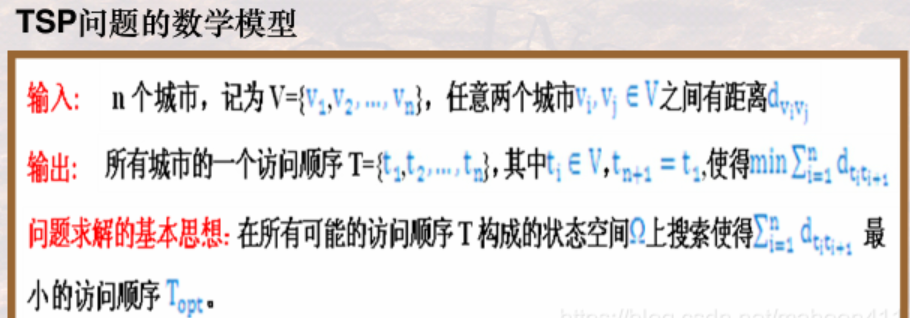三、差分进化算法简介
1 前言
在遗传、选择和变异的作用下，自然界生物体优胜劣汰，不断由低级向高级进化和发展。人们注意到，适者生存的进化规律可以模式化，从而构成一些优化算法；近年来发展的进化计算类算法受到了广泛的关注。 差分进化算法(Differential Evolution， DE) 是一种新兴的进化计算技术 。它是由S torn等人于1995年提出的， 其最初的设想是用于解决切比雪夫多项式问题，后来发现它也是解决复杂优化问题的有 效技术。 差分进化算法是基于群体智能理论的优化算法，是通过群体内个体间的合作与竞争而产生的智能优化搜索算法。但相比于进化计算，它保留了基于种群的全局搜索策略，采用实数编码、基于差分的简单 变异操作和“一对一”的竞争生存策略，降低了进化计算操作的复杂性。同时，差分进化算法特有的记忆能力使其可以动态跟踪当前的搜索情况，以调整其搜索策略，它具有较强的全局收敛能力和稳健性， 且不需要借助问题的特征信息，适用于求解一些利用常规的数学规划方法很难求解甚至无法求解的复杂优化问题[2-5]。因此，差分进化算法作为一种高效的并行搜索算法，对其进行理论和应用研究具有重要的学术意义和工程价值。 目前，差分进化算法已经在许多领域得到了应用，如人工神经元网络、电力、机械设计、机器人、信号处理、生物信息、经济学、现代农业和运筹学等。然而，尽管差分进化算法获得了广泛研究，但相 对于其他进化算法而言，其研究成果相当分散，缺乏系统性，尤其在理论方面还没有重大突破。
2 差分进化算法理论 2.1差分进化算法原理 差分进化算法是一种随机的启发式搜索算法，简单易用，有较强的鲁棒性和全局寻优能力。它从数学角度看是一种随机搜索算法，从工程角度看是一种自适应的迭代寻优过程。除了具有较好的收敛性外，差分进化算法非常易于理解与执行，它只包含不多的几个控制参数，并且在整个迭代过程中，这些参数的值可以保持不变。差分进化算法是一种自组织最小化方法，用户只需很少的输入。它的关键思想与传统进化方法不同：传统方法是用预先确定的概率分布函数决定向量扰动；而差分进化算法的自组织程序利用种群中两个随机选择的不同向量来干扰一个现有向量，种群中的每一个向量都要进行干扰。差分进化算法利用一个向量种群，其中种群向量的随机扰动可独立进行，因此是并行的。如果新向量对应函数值的代价比它们的前辈代价小，它们将取代前辈向量。 同其他进化算法一样，差分进化算法也是对候选解的种群进行操作，但其种群繁殖方案与其他进化算法不同：它通过把种群中两个成员之间的加权差向量加到第三个成员上来产生新的参数向量，该操作 称为“变异”； 然后将变异向量的参数与另外预先确定的目标向量参数按一定规则混合来产生试验量，该操作称为“交叉”；最后，若试验向量的代价函数比目标向量的代价函数低，试验向量就在下一代中代替目标向量，该操作称为“选择”。种群中所有成员必须当作目标向量进行一次这样的操作，以便在下一代中出现相同个数竞争者。 在进化过程中对每一代的最佳参数向量都进行评价，以记录最小化过程。这样利用随机偏差扰动产生新个体的方式，可以获得一个收敛性非常好的结果，引导搜索过程向全局最优解逼近[6-7]。
2.2差分进化算法的特点 差分进化算法从提出到现在，在短短二十几年内人们对其进行了广泛的研究并取得了成功的应用。该算法主要有如下特点： (1)结构简单，容易使用。差分进化算法主要通过差分变异算子来进行遗传操作，由于该算子只涉及向量的加减运算，因此很容易实现；该算法采用概率转移规则，不需要确定性的规则。此外，差分进化算法的控制参数少，这些参数对算法性能的影响已经得到一定的研究，并得出了一些指导性的建议，因而可以方便使用人员根据问题选择较优的参数设置。 (2)性能优越。差分进化算法具有较好的可靠性、高效性和鲁棒性，对于大空间、非线性和不可求导的连续问题，其求解效率比其他进化方法好，而且很多学者还在对差分进化算法继续改良，以不断提高其性能。 (3)自适应性。差分进化算法的差分变异算子可以是固定常数，也可以具有变异步长和搜索方向自适应的能力，根据不同目标函数进行自动调整，从而提高搜索质量。 (4)差分进化算法具有内在的并行性，可协同搜索，具有利用个体局部信息和群体全局信息指导算法进一步搜索的能力。在同样精度要求下，差分进化算法具有更快的收敛速度。 (5)算法通用，可直接对结构对象进行操作，不依赖于问题信息，不存在对目标函数的限定。差分进化算法操作十分简单，易于编程实现，尤其利于求解高维的函数优化问题。
3 差分进化算法种类 3.1基本差分进化算法 基本差分进化算法的操作程序如下： (1)初始化； (2)变异； (3)交叉； (4)选择； (5)边界条件处理。 初始化 差分进化算法利用NP个维数为D的实数值参数向量，将它们作为每 一代的种群，每个个体表示为：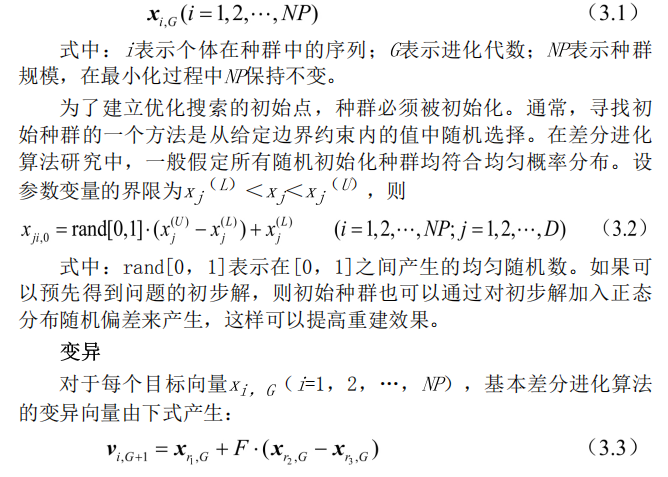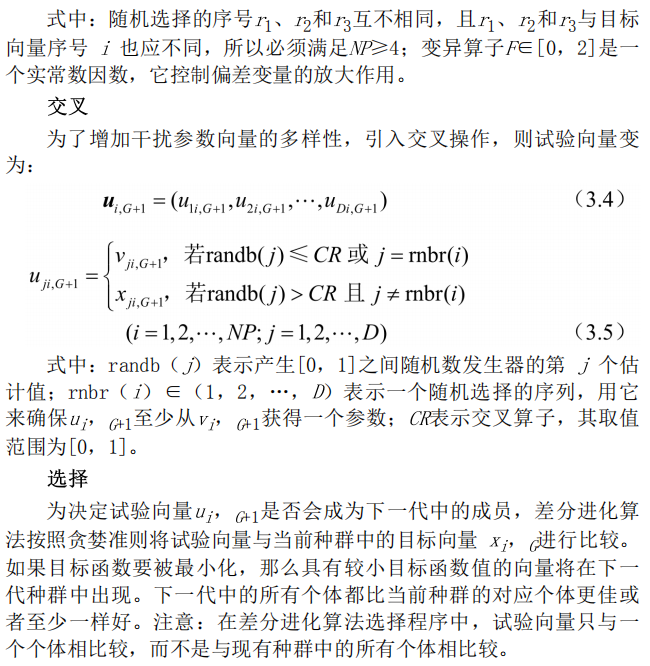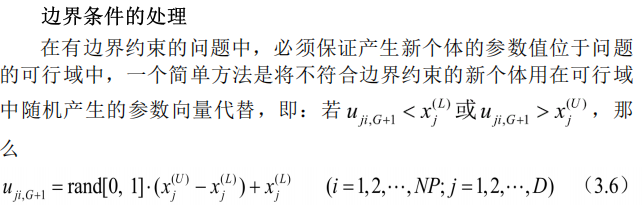另外一个方法是进行边界吸收处理，即将超过边界约束的个体值设置为临近的边界值。
3.2差分进化算法的其他形式 上面阐述的是最基本的差分进化算法操作程序，实际应用中还发展了差分进化算法的几个变形形式，用符号DE/x/y/z加以区分，其中：x限定当前被变异的向量是“随机的”或“最佳的”；y是所利用的差向量的个数；z指示交叉程序的操作方法。前面叙述的交叉操作表示为“bin”。利用这个表示方法， 基本差分进化算法策略可描述为DE/rand/1/bin。 还有其他形式[5，如：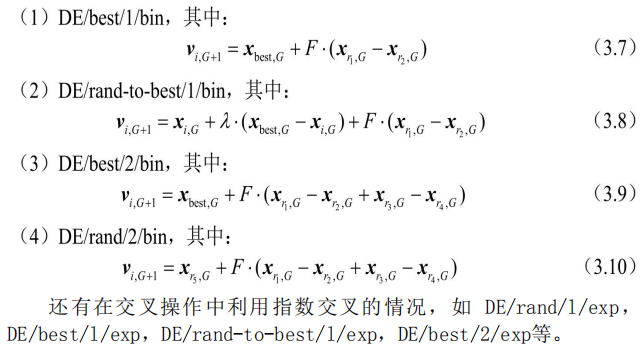3.3改进的差分进化算法 自适应差分进化算法 作为一种高效的并行优化算法，差分进化算法发展很快，出现了很多改进的差分进化算法。下面介绍一种具有自适应算子的差分进化算法.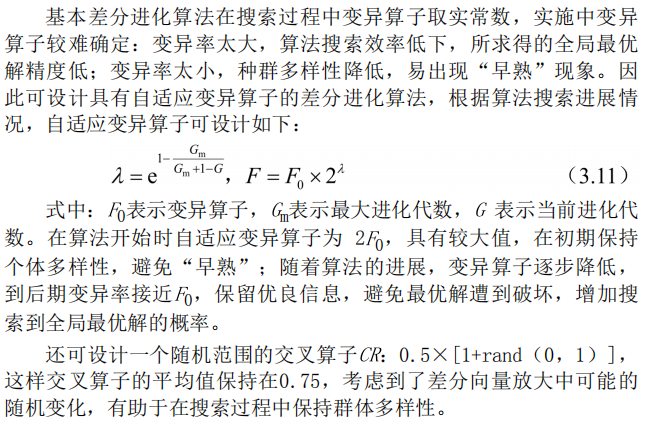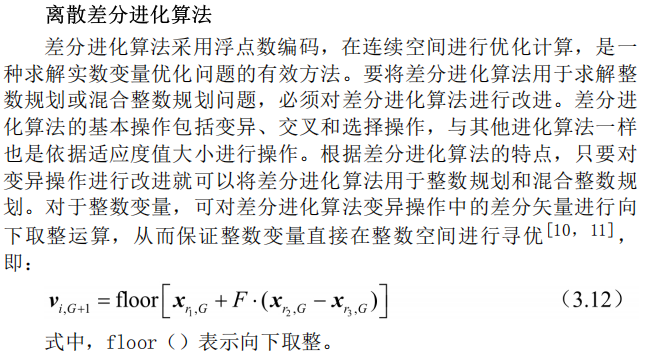4差分进化算法流程 差分进化算法采用实数编码、基于差分的简单变异操作和“一对一”的竞争生存策略，其具体步骤如下： (1)确定差分进化算法的控制参数和所要采用的具体策略。差分进化算法的控制参数包括：种群数量、变异算子、交叉算子、最大进化代数、终止条件等。 (2)随机产生初始种群，进化代数k=1。 (3)对初始种群进行评价，即计算初始种群中每个个体的目标函数值。 (4)判断是否达到终止条件或达到最大进化代数：若是，则进化终止，将此时的最佳个体作为解输出；否则，继续下一步操作。 (5)进行变异操作和交叉操作，对边界条件进行处理，得到临时种群。 (6)对临时种群进行评价，计算临时种群中每个个体的目标函数值。 (7)对临时种群中的个体和原种群中对应的个体，进行“一对-”的选择操作，得到新种群。 (8)进化代数k=k+1，转步骤(4)。 差分进化算法运算流程如图3.1所示。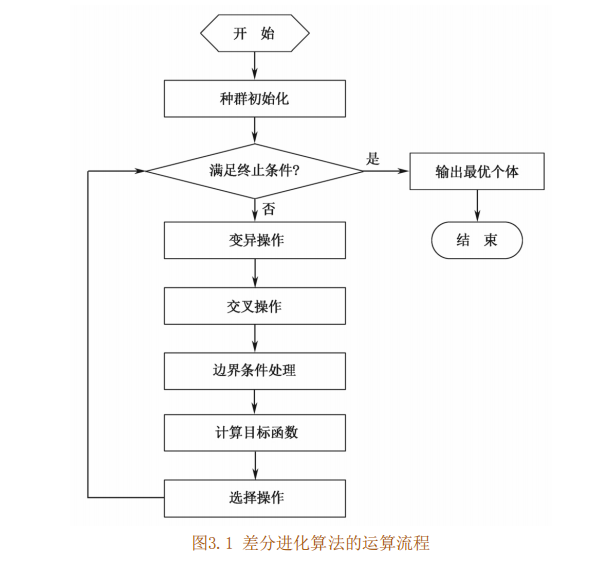5关键参数的说明 控制参数对一个全局优化算法的影响是很大的，差分进化算法的控制变量选择也有一些经验规则。
种群数量NP 一般情况下，种群的规模AP越大，其中的个体就越多，种群的多样性也就越好，寻优的能力也就越强，但也因此增加了计算的难度。所以，NP不能无限取大。根据经验，种群数量NP的合理选择在5D~ 10D之间，必须满足NP≥4，以确保差分进化算法具有足够的不同的变异向量。
变异算子F 变异算子FE[0，2]是一个实常数因数，它决定偏差向量的放大比例。变异算子熨小，则可能造成算法“早熟”。随着/值的增大，防止算法陷入局部最优的能力增强，但当F>1时，想要算法快速收敛到最优值会变得十分不易；这是由于当差分向量的扰动大于两个个体之间的距离时，种群的收敛性会变得很差。目前的研究表明，F小于0.4和大于1的值仅偶尔有效，/=0.5通常是一个较好的初始选择。若种 群过早收敛，那么F或NP应该增大。
交叉算子CR 交叉算子CR是一个范围在[0，1]内的实数，它控制着一个试验向量参数来自于随机选择的变异向量而不是原来向量的概率。交叉算子CK越大，发生交叉的可能性就越大。CR的一个较好的选择是0.1，但 较大的CK通常会加速收敛，为了看看是否可能获得一个快速解，可以先尝试CR=0.9或CF=1.0.
最大进化代数G 最大进化代数6是表示差分进化算法运行结束条件的一个参数，表示差分进化算法运行到指定的进化代数之后就停止运行，并将当前群体中的最佳个体作为所求问题的最优解输出。一般，6取100~500。
终止条件 除最大进化代数可作为差分进化算法的终止条件外，还可以增加其他判定准则。一般当目标函数值小于阈值时程序终止，阈值常选为10-6。上述参数中，F、CR与NP一样，在搜索过程中是常数，一般F和CR影响搜索过程的收敛速度和稳健性，它们的优化值不仅依赖于目标函数的特性，还与NP有关。通常可通过对不同值做一些试验之后，利用试验和结果误差找到F、CR和NP的合适值。
四、部分源代码
close all
clear
clc

%edit by zhang
% 2014-3-15

city=[1304,2312;3639,1315;4177,2244;3712,1399;3488,1535;3326,1556;...
3238,1229;4196,1004;4312,790;4386,570;3007,1970;2562,1756;2788,1491;...
2381,1676;1332,695;3715,1678;3918,2179;4061,2370;3780,2212;3676,2578;4029,2838;...
4263,2931;3429,1908;3507,2367;3394,3201;3439,3201;2935,3240;3140,3550;2545,2357;2778,2826;2370,2975];

city_num=size(city,1);
%计算两两城市之间的距离
for i=1:city_num
for j=1:city_num
distance(i,j)=sqrt((city(i,1)-city(j,1))^2 + (city(i,2)-city(j,2))^2);
end
end
%相比于其他算法，迭代次数略长
NP=20;
NG=2000;
F=0.6;
CR=0.5;

pop=zeros(NP,city_num);
x_old=zeros(NP,city_num);
x_new=zeros(NP,city_num);
pi_old=zeros(NP,city_num);
pi_new=zeros(NP,city_num);

for i=1:NP
for j=1:city_num
pop(i,j)=5*rand();
end
end

k=1;

x_old=pop;

while k<=NG
%将实数编码转换成工件顺序编码
for i=1:NP
x_old_decode(i,:)=LOVdecode(x_old(i,:));
end
%----find the best value--------------%
ind_best  = x_old(1,:);
for i=2:NP
pi_best=LOVdecode(ind_best);

if CalLength(distance,pi_best)>CalLength(distance,x_old_decode(i,:))
ind_best = x_old(i,:);
end
end

BestFit=CalLength(distance , LOVdecode(ind_best));%最短时间
Best=LOVdecode(ind_best);%最佳路径
%%--------mutaiton---------------------%
for i=1:NP
R=randperm(NP);
r1=R(1);
r2=R(2);
r3=R(3);

if i==r1
r1=R(4);
else if i==r2
r2=R(4);
else if i==r3
r3=R(4);
end
end
end

x_old = x_new;
k=k+1;
end
BSF=Best;
BestL=BestFit;
%绘制路径图
for i=1:city_num-1
plot([city(BSF(i),1),city(BSF(i+1),1)],[city(BSF(i),2),city(BSF(i+1),2)],'bo-');
hold on;
end
plot([city(BSF(city_num),1),city(BSF(1),1)],[city(BSF(city_num),2),city(BSF(1),2)],'ro-');
title('差分进化TSP')

disp('最佳路径');
disp(BSF);
disp('最短路径长度');
disp(BestL)

五、运行结果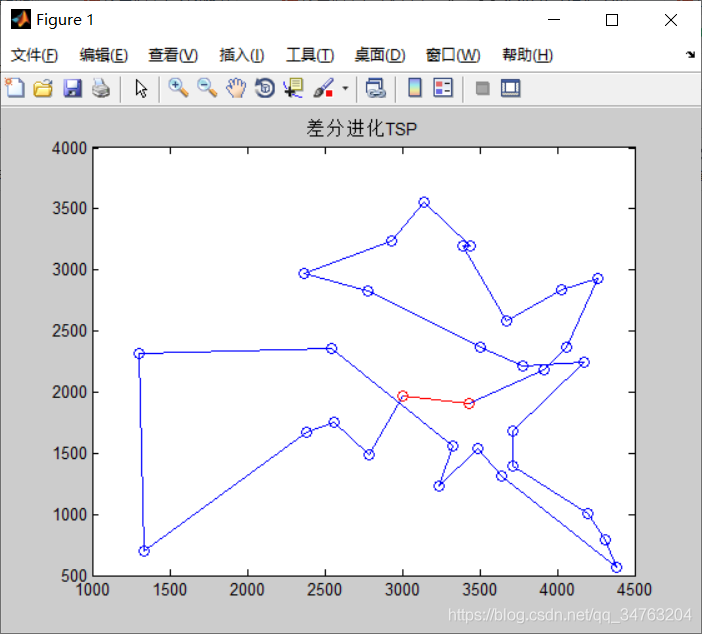六、matlab版本及参考文献
1 matlab版本 2014a
2 参考文献  包子阳,余继周,杨杉.智能优化算法及其MATLAB实例（第2版）[M].电子工业出版社，2016. 张岩,吴水根.MATLAB优化算法源代码[M].清华大学出版社，2017.
展开全文开发语言
• 一、简介 1 前言 在遗传、选择和变异的作用下，自然界生物体优胜劣汰，不断由低级向高级进化和发展。人们注意到，适者生存的进化规律可以模式...差分进化算法是基于群体智能理论的优化算法，是通过群体内个体间的合作与
一、获取代码方式
获取代码方式1： 通过订阅紫极神光博客付费专栏，凭支付凭证，私信博主，可获得此代码。
获取代码方式2： 通过紫极神光博客主页开通CSDN会员，凭支付凭证，私信博主，可获得此代码。
获取代码方式3： 完整代码已上传我的资源：【优化求解】基于matlab差分进化算法求解函数极值问题【含Matlab源码 1199期】
备注：开通CSDN会员，仅只能免费获得1份代码（有效期为开通日起，三天内有效）； 订阅紫极神光博客付费专栏，可免费获得2份代码（有效期为订阅日起，三天内有效）；
二、差分进化算法简介
1 前言
在遗传、选择和变异的作用下，自然界生物体优胜劣汰，不断由低级向高级进化和发展。人们注意到，适者生存的进化规律可以模式化，从而构成一些优化算法；近年来发展的进化计算类算法受到了广泛的关注。 差分进化算法(Differential Evolution， DE) 是一种新兴的进化计算技术 。它是由S torn等人于1995年提出的， 其最初的设想是用于解决切比雪夫多项式问题，后来发现它也是解决复杂优化问题的有 效技术。 差分进化算法是基于群体智能理论的优化算法，是通过群体内个体间的合作与竞争而产生的智能优化搜索算法。但相比于进化计算，它保留了基于种群的全局搜索策略，采用实数编码、基于差分的简单 变异操作和“一对一”的竞争生存策略，降低了进化计算操作的复杂性。同时，差分进化算法特有的记忆能力使其可以动态跟踪当前的搜索情况，以调整其搜索策略，它具有较强的全局收敛能力和稳健性， 且不需要借助问题的特征信息，适用于求解一些利用常规的数学规划方法很难求解甚至无法求解的复杂优化问题[2-5]。因此，差分进化算法作为一种高效的并行搜索算法，对其进行理论和应用研究具有重要的学术意义和工程价值。 目前，差分进化算法已经在许多领域得到了应用，如人工神经元网络、电力、机械设计、机器人、信号处理、生物信息、经济学、现代农业和运筹学等。然而，尽管差分进化算法获得了广泛研究，但相 对于其他进化算法而言，其研究成果相当分散，缺乏系统性，尤其在理论方面还没有重大突破。
2 差分进化算法理论 2.1差分进化算法原理 差分进化算法是一种随机的启发式搜索算法，简单易用，有较强的鲁棒性和全局寻优能力。它从数学角度看是一种随机搜索算法，从工程角度看是一种自适应的迭代寻优过程。除了具有较好的收敛性外，差分进化算法非常易于理解与执行，它只包含不多的几个控制参数，并且在整个迭代过程中，这些参数的值可以保持不变。差分进化算法是一种自组织最小化方法，用户只需很少的输入。它的关键思想与传统进化方法不同：传统方法是用预先确定的概率分布函数决定向量扰动；而差分进化算法的自组织程序利用种群中两个随机选择的不同向量来干扰一个现有向量，种群中的每一个向量都要进行干扰。差分进化算法利用一个向量种群，其中种群向量的随机扰动可独立进行，因此是并行的。如果新向量对应函数值的代价比它们的前辈代价小，它们将取代前辈向量。 同其他进化算法一样，差分进化算法也是对候选解的种群进行操作，但其种群繁殖方案与其他进化算法不同：它通过把种群中两个成员之间的加权差向量加到第三个成员上来产生新的参数向量，该操作 称为“变异”； 然后将变异向量的参数与另外预先确定的目标向量参数按一定规则混合来产生试验量，该操作称为“交叉”；最后，若试验向量的代价函数比目标向量的代价函数低，试验向量就在下一代中代替目标向量，该操作称为“选择”。种群中所有成员必须当作目标向量进行一次这样的操作，以便在下一代中出现相同个数竞争者。 在进化过程中对每一代的最佳参数向量都进行评价，以记录最小化过程。这样利用随机偏差扰动产生新个体的方式，可以获得一个收敛性非常好的结果，引导搜索过程向全局最优解逼近[6-7]。
2.2差分进化算法的特点 差分进化算法从提出到现在，在短短二十几年内人们对其进行了广泛的研究并取得了成功的应用。该算法主要有如下特点： (1)结构简单，容易使用。差分进化算法主要通过差分变异算子来进行遗传操作，由于该算子只涉及向量的加减运算，因此很容易实现；该算法采用概率转移规则，不需要确定性的规则。此外，差分进化算法的控制参数少，这些参数对算法性能的影响已经得到一定的研究，并得出了一些指导性的建议，因而可以方便使用人员根据问题选择较优的参数设置。 (2)性能优越。差分进化算法具有较好的可靠性、高效性和鲁棒性，对于大空间、非线性和不可求导的连续问题，其求解效率比其他进化方法好，而且很多学者还在对差分进化算法继续改良，以不断提高其性能。 (3)自适应性。差分进化算法的差分变异算子可以是固定常数，也可以具有变异步长和搜索方向自适应的能力，根据不同目标函数进行自动调整，从而提高搜索质量。 (4)差分进化算法具有内在的并行性，可协同搜索，具有利用个体局部信息和群体全局信息指导算法进一步搜索的能力。在同样精度要求下，差分进化算法具有更快的收敛速度。 (5)算法通用，可直接对结构对象进行操作，不依赖于问题信息，不存在对目标函数的限定。差分进化算法操作十分简单，易于编程实现，尤其利于求解高维的函数优化问题。
3 差分进化算法种类 3.1基本差分进化算法 基本差分进化算法的操作程序如下： (1)初始化； (2)变异； (3)交叉； (4)选择； (5)边界条件处理。 初始化 差分进化算法利用NP个维数为D的实数值参数向量，将它们作为每 一代的种群，每个个体表示为：另外一个方法是进行边界吸收处理，即将超过边界约束的个体值设置为临近的边界值。
3.2差分进化算法的其他形式 上面阐述的是最基本的差分进化算法操作程序，实际应用中还发展了差分进化算法的几个变形形式，用符号DE/x/y/z加以区分，其中：x限定当前被变异的向量是“随机的”或“最佳的”；y是所利用的差向量的个数；z指示交叉程序的操作方法。前面叙述的交叉操作表示为“bin”。利用这个表示方法， 基本差分进化算法策略可描述为DE/rand/1/bin。 还有其他形式[5，如：3.3改进的差分进化算法 自适应差分进化算法 作为一种高效的并行优化算法，差分进化算法发展很快，出现了很多改进的差分进化算法。下面介绍一种具有自适应算子的差分进化算法.4差分进化算法流程 差分进化算法采用实数编码、基于差分的简单变异操作和“一对一”的竞争生存策略，其具体步骤如下： (1)确定差分进化算法的控制参数和所要采用的具体策略。差分进化算法的控制参数包括：种群数量、变异算子、交叉算子、最大进化代数、终止条件等。 (2)随机产生初始种群，进化代数k=1。 (3)对初始种群进行评价，即计算初始种群中每个个体的目标函数值。 (4)判断是否达到终止条件或达到最大进化代数：若是，则进化终止，将此时的最佳个体作为解输出；否则，继续下一步操作。 (5)进行变异操作和交叉操作，对边界条件进行处理，得到临时种群。 (6)对临时种群进行评价，计算临时种群中每个个体的目标函数值。 (7)对临时种群中的个体和原种群中对应的个体，进行“一对-”的选择操作，得到新种群。 (8)进化代数k=k+1，转步骤(4)。 差分进化算法运算流程如图3.1所示。5关键参数的说明 控制参数对一个全局优化算法的影响是很大的，差分进化算法的控制变量选择也有一些经验规则。
种群数量NP 一般情况下，种群的规模AP越大，其中的个体就越多，种群的多样性也就越好，寻优的能力也就越强，但也因此增加了计算的难度。所以，NP不能无限取大。根据经验，种群数量NP的合理选择在5D~ 10D之间，必须满足NP≥4，以确保差分进化算法具有足够的不同的变异向量。
变异算子F 变异算子FE[0，2]是一个实常数因数，它决定偏差向量的放大比例。变异算子熨小，则可能造成算法“早熟”。随着/值的增大，防止算法陷入局部最优的能力增强，但当F>1时，想要算法快速收敛到最优值会变得十分不易；这是由于当差分向量的扰动大于两个个体之间的距离时，种群的收敛性会变得很差。目前的研究表明，F小于0.4和大于1的值仅偶尔有效，/=0.5通常是一个较好的初始选择。若种 群过早收敛，那么F或NP应该增大。
交叉算子CR 交叉算子CR是一个范围在[0，1]内的实数，它控制着一个试验向量参数来自于随机选择的变异向量而不是原来向量的概率。交叉算子CK越大，发生交叉的可能性就越大。CR的一个较好的选择是0.1，但 较大的CK通常会加速收敛，为了看看是否可能获得一个快速解，可以先尝试CR=0.9或CF=1.0.
最大进化代数G 最大进化代数6是表示差分进化算法运行结束条件的一个参数，表示差分进化算法运行到指定的进化代数之后就停止运行，并将当前群体中的最佳个体作为所求问题的最优解输出。一般，6取100~500。
终止条件 除最大进化代数可作为差分进化算法的终止条件外，还可以增加其他判定准则。一般当目标函数值小于阈值时程序终止，阈值常选为10-6。上述参数中，F、CR与NP一样，在搜索过程中是常数，一般F和CR影响搜索过程的收敛速度和稳健性，它们的优化值不仅依赖于目标函数的特性，还与NP有关。通常可通过对不同值做一些试验之后，利用试验和结果误差找到F、CR和NP的合适值。
三、案例及完整源代码
1 案例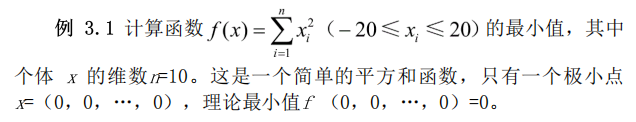2 完整代码
%%%%%%%%%%%%%%%%%差分进化算法求函数极值%%%%%%%%%%%%%%%%%%
%%%%%%%%%%%%%%%%%%%%%%%%%初始化%%%%%%%%%%%%%%%%%%%%%%%%%
clear all;                            %清除所有变量
close all;                            %清图
clc;                                  %清屏
NP=50;                                %个体数目
D=10;                                 %变量的维数
G=200;                                %最大进化代数
F0=0.4;                               %初始变异算子
CR=0.1;                               %交叉算子
Xs=20;                                %上限
Xx=-20;                               %下限
yz=10^-6;                             %阈值
%%%%%%%%%%%%%%%%%%%%%%%%%赋初值%%%%%%%%%%%%%%%%%%%%%%%%
x=zeros(D,NP);                        %初始种群
v=zeros(D,NP);                        %变异种群
u=zeros(D,NP);                        %选择种群
x=rand(D,NP)*(Xs-Xx)+Xx;              %赋初值
%%%%%%%%%%%%%%%%%%%%计算目标函数%%%%%%%%%%%%%%%%%%%%
for m=1:NP
Ob(m)=func1(x(:,m));
end
trace(1)=min(Ob);
%%%%%%%%%%%%%%%%%%%%%%%差分进化循环%%%%%%%%%%%%%%%%%%%%%
for gen=1:G
%%%%%%%%%%%%%%%%%%%%%%变异操作%%%%%%%%%%%%%%%%%%%%%%
%%%%%%%%%%%%%%%%%%%自适应变异算子%%%%%%%%%%%%%%%%%%%
lamda=exp(1-G/(G+1-gen));
F=F0*2^(lamda);
%%%%%%%%%%%%%%%%%r1,r2,r3和m互不相同%%%%%%%%%%%%%%%%
for m=1:NP
r1=randi([1,NP],1,1);
while (r1==m)
r1=randi([1,NP],1,1);
end
r2=randi([1,NP],1,1);
while (r2==m)|(r2==r1)
r2=randi([1,NP],1,1);
end
r3=randi([1,NP],1,1);
while (r3==m)|(r3==r1)|(r3==r2)
r3=randi([1,NP],1,1);
end
v(:,m)=x(:,r1)+F*(x(:,r2)-x(:,r3));
end
%%%%%%%%%%%%%%%%%%%%%%交叉操作%%%%%%%%%%%%%%%%%%%%%%%
r=randi([1,D],1,1);
for n=1:D
cr=rand(1);
if (cr<=CR)|(n==r)
u(n,:)=v(n,:);
else
u(n,:)=x(n,:);
end
end
%%%%%%%%%%%%%%%%%%%边界条件的处理%%%%%%%%%%%%%%%%%%%%%
for n=1:D
for m=1:NP
if (u(n,m)<Xx)|(u(n,m)>Xs)
u(n,m)=rand*(Xs-Xx)+Xx;
end
end
end
%%%%%%%%%%%%%%%%%%%%%%选择操作%%%%%%%%%%%%%%%%%%%%%%%
for m=1:NP
Ob1(m)=func1(u(:,m));
end
for m=1:NP
if Ob1(m)<Ob(m)
x(:,m)=u(:,m);
end
end
for m=1:NP
Ob(m)=func1(x(:,m));
end
trace(gen+1)=min(Ob);
if min(Ob(m))<yz
break
end
end
[SortOb,Index]=sort(Ob);
x=x(:,Index);
X=x(:,1);                              %最优变量
Y=min(Ob);                             %最优值
%%%%%%%%%%%%%%%%%%%%%%%%%画图%%%%%%%%%%%%%%%%%%%%%%%%%%
figure
plot(trace);
xlabel('迭代次数')
ylabel('目标函数值')
title('适应度进化曲线')


%%%%%%%%%%%%%%%%%%%%%%%适应度函数%%%%%%%%%%%%%%%%%%%%%%%%
function result=func1(x)
summ=sum(x.^2);
result=summ;

四、解题过程及运行结果
1 解题过程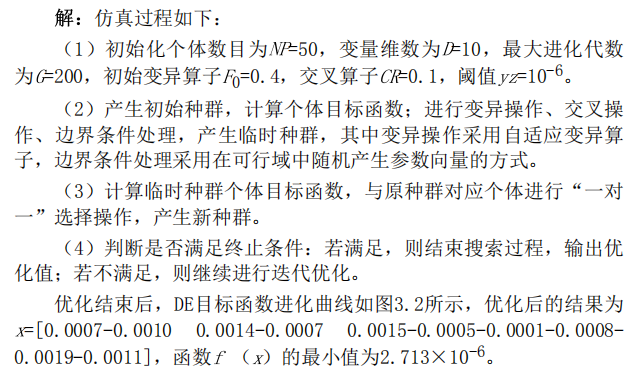2 运行结果五、matlab版本及参考文献
1 matlab版本 2014a
2 参考文献 《智能优化算法及其MATLAB实例（第2版）》包子阳 余继周 杨杉著 电子工业出版社
展开全文• MATLAB程序实现差分进化算法，程序包含5个文件，分别为主程序、初始化种群、适应度函数（选择）、交叉、变异。程序示例为设计一阶控制器使离散传递函数(z-1)(z+0.3)/z(z-2)(z-0.5)稳定。
• 根据Rainer Storn和Kenneth Price在1997年发表的文章Differential Evolution - A Simple and Efficient Heuristic for Global Optimization over Continuous制作，内附原文及代码。
• ## 差分进化算法之Matlab实现

千次阅读 多人点赞 2019-03-25 18:04:24
差分进化算法是模拟自然界生物种群以“优胜劣汰，适者生存”为原则的进化发展规律而形成的一种随机启发式搜索算法。其保留了基于种群的全局搜索策略，采用实数编码，基于差分的简单变异操作和一对一的竞争生存策略，...
一、介绍 差分进化算法是模拟自然界生物种群以“优胜劣汰，适者生存”为原则的进化发展规律而形成的一种随机启发式搜索算法。其保留了基于种群的全局搜索策略，采用实数编码，基于差分的简单变异操作和一对一的竞争生存策略，比遗传算法更简单。同时，差分进化算法独特的记忆能力使其可以动态的跟踪当前的搜索情况，及时调整搜索测量，因此具有较强的全局收敛能力。 目前为止，差分进化算法已经成为一种求解非线性，不可微，多极值和高维复杂函数的一种极其有效的方法。 在优化设计中，差分进化算法与传统的算法相比，具有以下特点： 1.差分进化算法从一个群体即多个点而不是从一个点开始搜索，这也是算法能够以较大的概率找到整体最优解的原因。 2.算法的进化准则是基于适应性信息的，不需要其他的辅助性信息，如要求函数可导，连续等。 3. 差分进化算法具有内在的并行性，适用于大规模并行分布处理，减小时间成本开销。 但缺点为： 1.算法后期个体之间的差异性减小，收敛速度慢，易陷入局部最优。 2.没有利用个体的先验知识，可能较多的迭代次数才能收敛到全局最优 算法框架：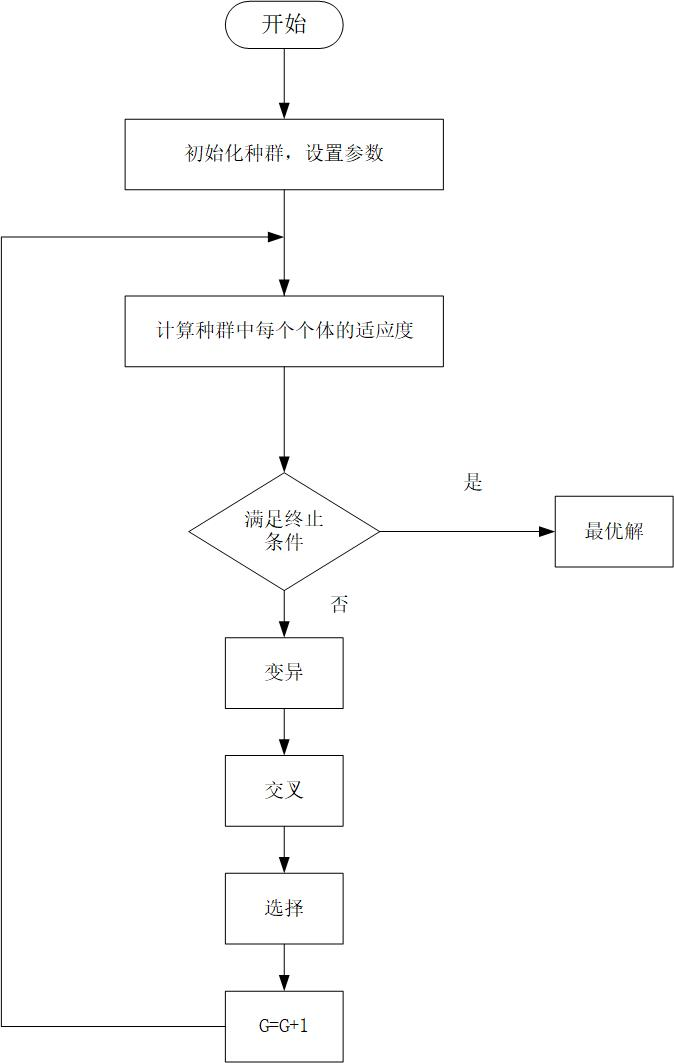(1)群体初始化 在n维空间里随机产生满足约束条件的M个个体

x

i

j

(

0

)

=

x

i

j

m

i

n

+

(

x

i

j

m

a

x

−

x

i

j

m

i

n

)

∗

r

a

n

d

(

0

,

1

)

x_{ij}(0)=x_{ij_{min}}+(x_{ij_{max}}-x_{ij_{min}})*rand(0,1)

其中，

x

i

j

m

a

x

,

x

i

j

m

i

n

x_{ij_{max}},x_{ij_{min}}

表示第

j

j

个染色体的上下界。 (2) 变异 从群体中随机选择三个个体

x

p

1

,

x

p

2

,

x

p

3

x_{p1},x_{p2},x_{p3}

且要求

i

≠

p

1

≠

p

2

≠

p

3

i\neq p1\neq p2\neq p3

,则：

h

i

j

(

t

+

1

)

=

x

p

1

j

(

t

)

+

F

∗

(

x

p

2

j

(

t

)

−

x

p

3

j

(

t

)

)

h_{ij}(t+1)=x_{p1j}(t)+F*(x_{p2j}(t)-x_{p3j}(t))

如果没有局部优化的问题，变异操作为：

h

i

j

(

t

+

1

)

=

x

b

j

(

t

)

+

F

∗

(

x

p

2

j

(

t

)

−

x

p

3

j

(

t

)

)

h_{ij}(t+1)=x_{bj}(t)+F*(x_{p2j}(t)-x_{p3j}(t))

其中，

x

p

2

j

(

t

)

−

x

p

3

j

(

t

)

x_{p2j}(t)-x_{p3j}(t)

为差异化向量，是差分进化算法的关键；F为变异因子；P1，P2,P3为随机整数，表示个体在种群中的序号；

x

b

j

x_{bj}

是当前种群中最好的个体，这一步借鉴了当前种群中最好的个体信息，可以大大加快收敛速度 (3) 交叉 交叉操作可以增加群体的多样性

v

i

j

(

t

+

1

)

=

{

h

i

j

(

t

+

1

)

,

r

a

n

d
&ThickSpace;

l

i

j

≤

C

R

h

x

i

j

(

t

)

,

r

a

n

d
&ThickSpace;

l

i

j

&gt;

C

R

v_{ij}(t+1)=\left\{ \begin{array}{c} h_{ij}(t+1),rand\; l_{ij} \leq CR\\ hx{ij}(t),rand\; l_{ij}&gt;CR\ \end{array}\right.

CR为交叉因子。 (4) 选择操作 为了确定

x

i

(

t

+

1

)

x_i(t+1)

是否成为下一代的成员，我们需要对目标向量和当前的向量的适应度值进行比较，具体由适应度函数决定：

x

i

(

t

+

1

)

=

{

v

i

(

t

+

1

)

,

f

(

v

i

1

(

t

+

1

)

,

.

.

.

v

i

n

(

t

+

1

)

)

&lt;

f

(

x

i

1

(

t

)

,

.

.

.

,

x

i

n

(

t

)

)

x

i

(

t

+

1

)

,

f

(

v

i

1

(

t

+

1

)

,

.

.

.

v

i

n

(

t

+

1

)

)

≥

f

(

x

i

1

(

t

)

,

.

.

.

,

x

i

n

(

t

)

)

x_{i}(t+1)=\left\{ \begin{array}{c} v_{i}(t+1),f(v_{i1}(t+1),...v_{in}(t+1)) &lt;f(x_{i1}(t),...,x_{in}(t))\\ x_{i}(t+1),f(v_{i1}(t+1),...v_{in}(t+1))\geq f(x_{i1}(t),...,x_{in}(t)) \ \end{array}\right.

通过反复执行步骤(2)到(4),直至达到最大的迭代次数。 二、参数设置 1.变异因子F 变异因子是控制种群多样性和收敛性的重要参数，当F值较小时，种群之间的差异度小，容易使得种群过早的收敛于局部最小值，当F过大时，容易跳出局部最优解，但是收敛速度会减慢。F一般在[0,2]之间取值。 2.交叉因子CR 交叉因子可以控制个体参数的各维对交叉的参与程度，全局搜索和局部搜索能力的平衡。CR越小，种群多样性减小，容易收敛于局部最优解。CR越大，收敛速度变快，但过大，扰动大于群体差异度时，会导致收敛变慢。CR一般取[0,1]之间。 3.群体规模Size Size一般为5D和10D之间，D时求解的维度。Size越大，获得最优解的概率越大，但计算时间增长。 4.迭代次数G G 一般作为近化过程的终止条件，G越大，最优解越精准。当然终止条件也可以由适应度函数给出。 三、matlab代码 以函数

f

(

x

,

y

)

=

−

20

e

−

0.2

（

x

2

+

y

2

）

/

2

−

e

(

c

o

s

2

π

x

+

c

o

s

2

π

y

)

/

2

+

e

f(x,y)=-20e^{-0.2\sqrt{（{x^2+y^2}）/2}}-e^{({cos2\pi x+cos2 \pi y})/2}+e

为例 三维图为：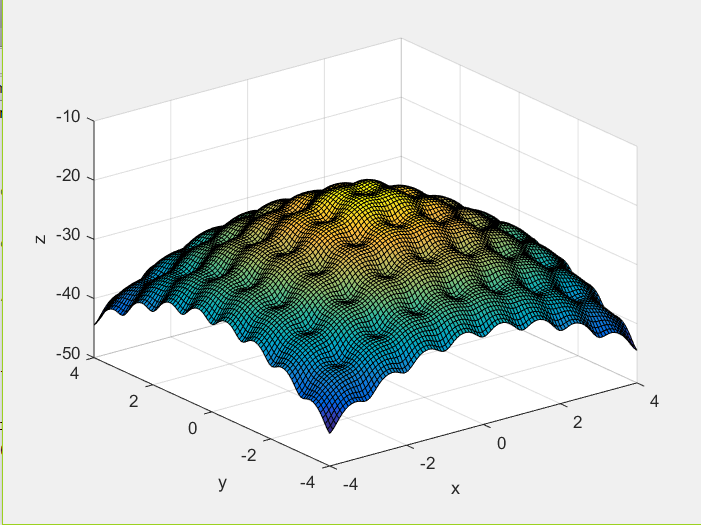可见该函数是多极值的。函数全局最优解为max(max(f(x,y)))=-19.2926，使用一般的算法，极易陷入局部的最优解。 使用差分进化算法，结果为-19.2523,与真实值十分的接近。 适应度函数变化曲线为：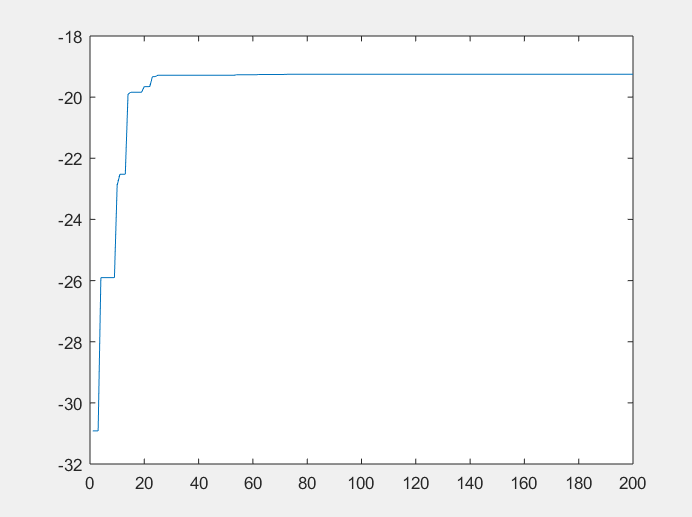matlab代码为：
% clear all;
% close all;
%
size=50;%群体个数
Codel=2;%所求的变量个数
MinX(1)=-5;%未知量范围
MinX(2)=-5;
MaxX(1)=5;
MaxX(2)=5;
G=200;%迭代次数
F=1.2;%变异因子[0 2]
cr=0.8;%交叉因子[0.6 0.9]
%初始化种群
for i=1:1:Codel
P(:,i)=MinX(i)+(MaxX(i)-MinX(i))*rand(size,1);
end

Best=P(1,:);%全局最优个体 之后不断更新
for i=2:size
if(fun_DE(P(i,1),P(i,2))>fun_DE(Best(1),Best(2)))
Best=P(i,:);
end
end
fi=fun_DE(Best(1),Best(2));%不是C语言 一定要记得给初始变量否则程序跑飞
%%进入循环直到满足精度要求或者迭代次数达到
for Kg=1:1:G
time(Kg)=Kg;
%第二步 变异
for i=1:size
r1=1;r2=1;r3=1;r4=1;%使得个体满足变异条件
while(r1==r2||r1==r3||r1==r4||r2==r3||r2==r4||r3==r4||r1==i||r2==i||r3==i||r4==i)
r1=ceil(size*rand(1));%大小匹配
r2=ceil(size*rand(1));
r3=ceil(size*rand(1));
r4=ceil(size*rand(1));
end
h(i,:)=P(r1,:)+F*(P(r2,:)-P(r3,:));
%h(i,:)=Best+F*(P(r2,:)-P(r3,:));
for j=1:Codel %检查是否越界
if(h(i,j)<MinX(j))
h(i,j)=MinX(j);
elseif(h(i,j)>MaxX(j))
h(i,j)=MaxX(j);
end
end
%交叉
for j=1:Codel
temper=rand(1);
if(temper<cr)
v(i,j)=h(i,j);
else
v(i,j)=P(i,j);
end
end
%选择
if(fun_DE(v(i,1),v(i,2))>fun_DE(P(i,1),P(i,2)))
P(i,:)=v(i,:);
end
if(fun_DE(P(i,1),P(i,2))>fi)
fi=fun_DE(P(i,1),P(i,2));
Best=P(i,:);
end
end
Best_f(Kg)=fun_DE(P(i,1),P(i,2));

end
fprintf('最优解结果为%f,%f',Best(1),Best(2));
fprintf('最大函数值为%f',Best_f(Kg));
plot(time,Best_f(time));

适应度函数
function J=fun_DE(x1,x2)
% J=100*(x1^2-x2)^2+(1-x1)^2;
J=-20*exp((0.2*sqrt((x1^2+x2^2)/2)))-exp((cos(2*pi*x1)+cos(2*pi*x2))/2)+exp(1);
end

展开全文• matlab开发-多目标优化差分进化算法。为多目标优化运行基本差分进化（DE）算法。
• 基于差分进化算法，求解函数最优值问题，并画出曲线。
• 优化的核心是差分进化算法。 但是，此包提供的代码远不止Differential Evolution 主页上提供的代码： http://www.icsi.berkeley.edu/~storn/code.html 以下是一些功能的列表： * 优化可以在多个内核/计算机上并行...
• 20世纪60年代初，美国Michigan大学的J.Holland教授借鉴与生物进化机制提出了自适应机器人学习，即成为后来广受人们关注的遗传算法；I.Rechenberg和L.J.Foge等人都面临着各自研究领域所遇到的复杂问题，受生物种群...
一、获取代码方式
获取代码方式1： 通过订阅紫极神光博客付费专栏，凭支付凭证，私信博主，可获得此代码。
获取代码方式2： 通过紫极神光博客主页开通CSDN会员，凭支付凭证，私信博主，可获得此代码。
获取代码方式3： 完整代码已上传我的资源：【数据分析】基于matlab差分进化算法函数优化分析【含Matlab源码 239期】
备注：开通CSDN会员，仅只能免费获得1份代码（有效期为开通日起，三天内有效）； 订阅紫极神光博客付费专栏，可免费获得2份代码（有效期为订阅日起，三天内有效）；
二、部分源代码
%% DE
%% 清空环境变量
clc,clear,close all
warning off
feature jit off
F0 = 0.5;  % 是变异率
Gm = 100;  % 最大迭代次数
Np = 100;  % 种群规模
CR = 0.9;  % 杂交参数
G = 1;     % 初始化代数
N = 10;    % 所求问题的维数，即待求解未知数个数
ge = zeros(1,Np);    % 各代的最优目标函数值
bestx = zeros(Np,N); % 各代的最优解
% 解范围
xmin = -5.12; % 下限
xmax = 5.12;  % 上限
% 产生初始种群
X0 = (xmax-xmin)*rand(Np,N)+xmin;
X = X0;
% 候选解初始化
X1new = zeros(Np,N);  % 初始化
X1_new = zeros(Np,N); % 初始化
X1 = zeros(Np,N);     % 初始化
value = zeros(1,Np);  % 初始化
while G<=Gm   % 迭代开始
disp(['迭代次数：  ',num2str(G)])

for i=1:Np
%产生j,k,p三个不同的数
a=1;b = Np;
dx = randperm(b-a+1)+a-1;
j=dx(1);k=dx(2);p=dx(3);
if j==i
j=dx(4);
elseif k==i
k=dx(4);
elseif p==i
p=dx(4);
end

% 变异算子
namd=exp(1-Gm/(Gm+1-G));
F=F0*2.^namd;

bon = X(p,:)+F*(X(j,:)-X(k,:)); % 个体更新
if (bon>xmin)&(bon<xmax)        % 防止变异超出边界
X1new(i,:)=bon;
else
X1new(i,:)=(xmax-xmin)*rand(1,N)+xmin;
end
end
% 杂交操作
for i=1:Np
if rand>CR   % 利用二项分布来交叉
X1_new(i,:)= X(i,:);
else
X1_new(i,:) = X1new(i,:);
end
end
% 竞争操作
for i=1:Np
if fitness(X1_new(i,:))<fitness(X(i,:))
X1(i,:)=X1_new(i,:);
else
X1(i,:)=X(i,:);
end
end
% 找出最小值
for i=1:Np
value(i)=fitness(X1(i,:));
end

三、运行结果四、matlab版本及参考文献
1 matlab版本 2014a
2 参考文献  由伟,刘亚秀.MATLAB数据分析教程[M].清华大学出版社，2020. 王岩,隋思涟.试验设计与MATLAB数据分析[M].清华大学出版社，2012.
展开全文• 自适应调节的差分进化算法matlab程序（原创）——RD-DE 算法在优化初期需要搜索能力更强的阶段采用[0,1]之间随机选择变异因子 F 以及交叉概率因子 CR 的策略；而在后期需要开发能力 更强的阶段则采用令 F 和 CR 单调...
• 基于matlab差分进化算法 可以测试一些函数的最小值
• 差分进化算法(Differential Evolution，DE)由Storn和Price于1995年首次提出。主要用于求解实数优化问题。该算法是一类基于群体的自适应全局优化算法，属于演化算法的一种，由于其具有结构简单、容易实现、收敛快速、...
• 差分进化算法概述 20世纪60年代初，美国 Michigan大学的.Holland教授借鉴与生物进化机制提出了自适应机器人学习，即成为后来广受人们关注的遗传算法； I Rechenberg和 LL.Foge等人都面临着各自研究领域所遇到的复杂...
• 差分进化算法可以快速求解方程的极值以及优化问题。
• 它实现了基于差分进化 (DE) 算法的基本多目标优化算法： “Storn, R., Price, K., 1997。差分进化：一种简单有效的连续空间全局优化启发式方法。全球优化杂志 11, 341 – 359。” 当一个目标被优化时，标准 DE ...
• 人工智能 车间调度 函数优化 参数自适应的差分进化算法 JADE matlab源代码
• ## 差分进化算法原理及matlab代码实现

万次阅读 多人点赞 2019-04-23 19:40:08
差分进化算法介绍： 在自然界中，遗传，变异，选择的作用，使得生物体优胜略汰，不断由低级向高级进化，人们发现适者生存这一规律可以模式化，从而构成一些列优化算法。差分进化算法就是从这种模式中产生的一种...
• 提供了自适应差分进化算法的代码，另包含测试函数集 %Reference: A. K. Qin, V. L. Huang, and P. N. Suganthan,“Differential evolution % algorithm with strategy adaptation for global numerical ...
• 基于差分进化算法的PID参数整定在XY平台跟踪控制中的应用，对相关算法及控制做出大概描述，给出PID参数整定的分析结果。
• 差分进化算法是一种全局最优算法，在差分演化算法当中需要注意的几个点为变异率F、交叉率Cr、基向量的选择r0,r1,r2的选择等问题。在研究差分演化算法的过程之中有许多的改进，对基向量的改进、变异概率、交叉概率的...机器学习...

# matlab的差分进化算法matlab 订阅